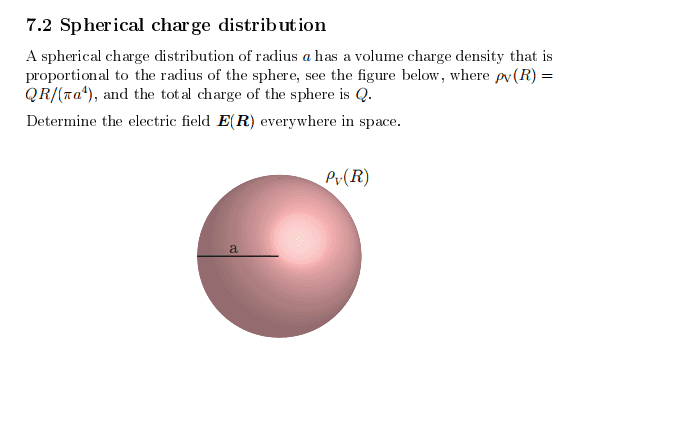# Electric field from spherical charge distribution

• JamMaster

#### JamMaster

Hello. I have a problem calculating the electric field from spherical charge distribution. The exercise is:
1. Homework Statement## Homework Equations

To solve the problem for $$0\le R < a$$ i tried 2 ways:
$$\vec{E} = \frac{\vec{a_R}}{4\pi\epsilon_0}\int_v\frac{1} {R^2}\rho dv$$
and the second way
$$V = \frac{1}{4\pi\epsilon_0}\int_v\frac{1}{R}\rho dv$$
and
$$\vec{E} = -\vec{\nabla}V$$

3. The Attempt at a Solution

Unfortunately, neither of the attempts worked.

Using first method, substituting the charge density from the text and using spherical coordinates to calculate the integral i got
$$\vec{E} = \frac{Q\vec{a_R}}{4\pi\epsilon_0a^4}\int_v\frac{1} {R}R^2\sin{\theta}dRd\theta d\phi = \frac{QR^2}{2\pi\epsilon_0a^4}\vec{a_R}$$
Which is wrong. The correct answer is very close though, where there is a 4 instead of 2 in the answer above. No matter how much i looked i couldn't find where i missed the constant.

Using the second method, i got
$$V = \frac{Q}{4\pi^2\epsilon_0a^4}\int_vR^2\sin{\theta}dRd\theta d\phi = \frac{QR^3}{3\pi\epsilon_0a^4}$$
Which gives the electric field
$$\vec{E} = -\vec{a_R} \frac{\partial{V}}{\partial{R}} = -\frac{QR^2}{\pi\epsilon_0a^4}\vec{a_R}$$
The above answer is even more wrong than the previous one.
I would really appreciate if someone could tell me what i have done wrong.

Hello JamMaster,Could you explain where your expression $$\vec{E} = \frac{\vec{a_R}}{4\pi\epsilon_0}\int_v\frac{1} {R^2}\rho dv$$comes from ? (I know it, and I can guess where you are derailing, so I ask you to show your steps in a bit more detail)

I take it ##\vec {a_R}## is a unit vector in the r-direction ? (don't forget to list it in part 1)

Hey and thank you for the answer.

Apparently i can't edit post so i can't update the first one.

Yes, $$\vec{a_R}$$ is a unit vector in R-direction, and R is the length of the same vector. The equation you named is simple a formula for calculating the electric field from a charge distribution. The formula comes from Coulombs law.

Yes, I know. As I said. But something's wrong. Could you please show your steps in a bit more detail.
Point being that the ##R## in the denominator is not the ##R## in ##dv##.
And that this is not a way to integrate a vector ##\vec {dE}##.
Make a little drawing and you'll see.

Anyway, if you are presented with an exercise like this, you are supposed to have mastered Coulomb already and know some much more effective tool to deal with this kind of problem.

--

Last edited:
•JamMaster
I see my mistake. Thank you a lot. I know this problem can be easily solved using Gauss Law, i just wanted to do it with all 3 methods.

This one is screaming "Gauss" all over.